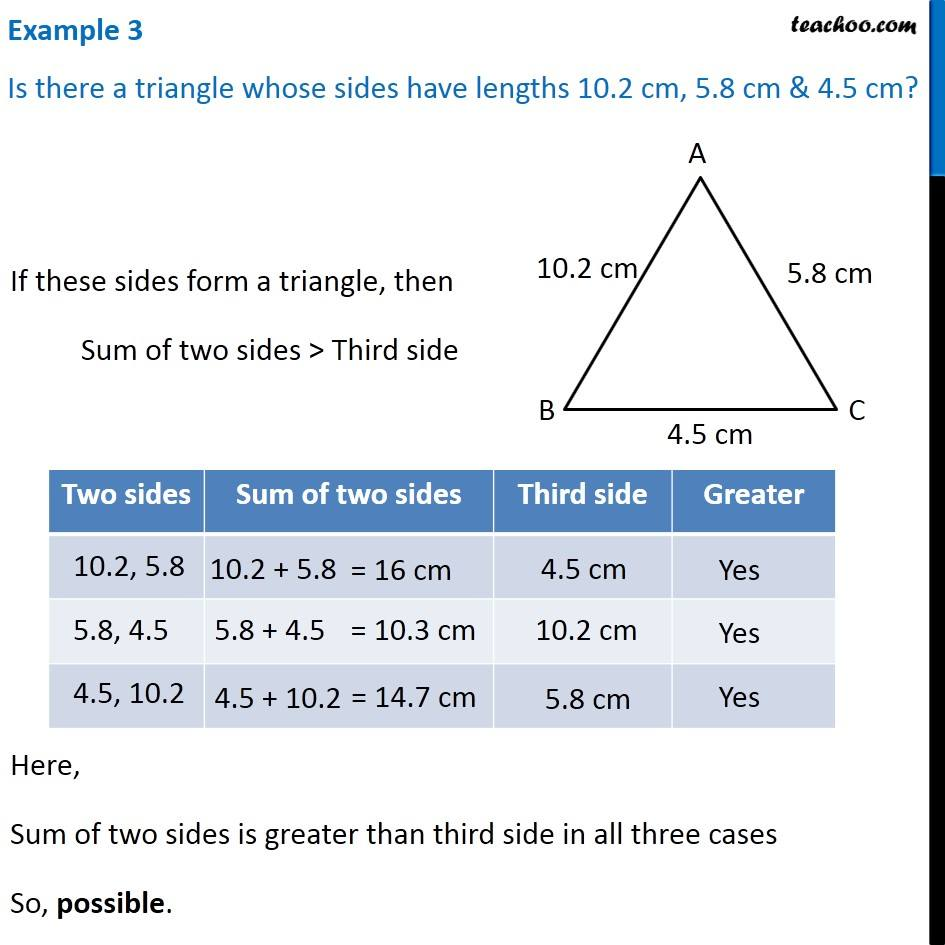1. Chapter 6 Class 7 Triangle and its Properties
2. Serial order wise
3. Examples

Transcript

Example 3 - Chapter 6 Class 7 Triangle and its Properties - Teachoo Is there a triangle whose sides have lengths 10.2 cm, 5.8 cm & 4.5 cm? If these sides form a triangle, then Sum of two sides > Third side If two sides are 10.2, 5.8 , Sum = 16 cm, which is greater than third side 4.5 cm 5.8, 4.5 , Sum = 10.3 cm, which is greater than third side 10.2 cm 4.5, 10.2 , Sum = 14.7 cm, which is greater than third side 5.8 cm Here, Sum of two sides is greater than third side in all three cases So, possible.